# Gas Laws Formulas & Physics For Scuba Diving

### Definition

The word hyperbaric means ‘greater than normal pressure’ and refers to gases such as oxygen and nitrogen that form most of the air we breathe. Hyperbaric therapy is the use of gases at greater than normal pressure for therapeutic reasons.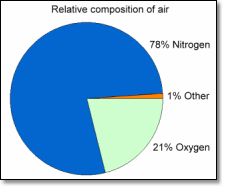Some points to remember are:

• The relationship between the pressure, volume and temperature of a gas.
• Air is made up of approximately 21% oxygen and 79% nitrogen.
• Under hyperbaric conditions, both oxygen and nitrogen can cause problems.
• Nitrogen is considered to be an ‘inert gas’ when discussing its effect on the body.

### Pressure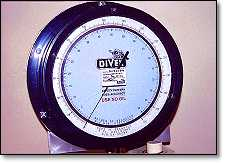Atmospheric pressure is caused by the weight of the air above, which in turn is the effect gravity has on the various gases in it. The more molecules there are in a given volume, the higher the pressure.

Above the surface of a body of water the force exerted is atmospheric (or barometric) pressure, which decreases with altitude. Under the surface, the weight of the water molecules causes hydrostatic pressure, which increases proportionally with the amount of water above (depth).

Because water is more dense than air, hydrostatic pressure in considerably greater than atmospheric. At a depth of 10 metres of seawater (msw) the force exerted is equivalent to the pressure exerted by the atmosphere at the surface. The actual pressure at this depth is equal to the hydrostatic plus atmospheric pressures. Therefore, the pressure at a depth of 10 msw equals 1 atmosphere plus the pressure of the atmosphere above, resulting in a total of 2 atmospheres. This is expressed as atmospheres absolute (ata).

Use the utility on the right to convert between atmospheres absolute (ata), gauge pressure (bar / psig) and depth (msw / fsw). Requires Javascript enabled.

Gas Laws Formulas & Physics For Scuba Diving

The important ones are:

1. Boyle’s law: Relates gas pressure to volume
2. Charles’ law: Relates gas pressure to temperature
3. Combined gas law: Combination of Boyle’s law and Charles’ law
4. Dalton’s law: Relates pressure of a mixed gas to the partial pressures of each gas in the mix
5. Henry’s law: Relates the gas dissolved in a fluid (like water) with the partial pressure of the gas in contact with the fluid.

### Boyle’s Law

Boyle’s law states that if the temperature remains constant, the volume of a fixed mass of a gas is inversely proportional to the pressure.

Gas volumes expand when the pressure decreases and contract when pressure increases.

Formulae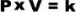(Where P is pressure, V is volume and k is a constant.)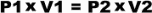(Where P1 and V1 are starting values, P2 and V2 are final values.)

Example

The volume in a diver’s lungs equals four litres of air at the surface. What would the volume of air be at 10msw if the diver held his breath? Firstly, the pressure at the surface equals 1ata. At 10msw it is 2ata.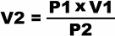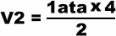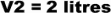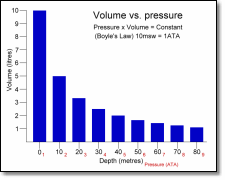The formula can be used to show that the volume will continue to fall as pressure increases – and that as pressure decreases the volume will increase. Along with Henry’s law, this is a key principle when considering diving and decompression sickness.

The graph to the right illustrates the relationship between pressure and volume (click on it for a larger view). Note that the change in volume is greatest nearer the surface.

### Charles’ Law

Charles’ law states that if the pressure remains constant, the volume of a fixed mass of a gas is directly proportional to the absolute temperature.

Formulae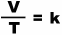(Where V is volume, T is absolute temperature in Kelvin and k is a constant.)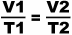(Where V1 and T1 are starting values, V2 and T2 are final values.)

Example

A balloon has a volume of 1L at 20°C, what is its volume at 50°C if it remains at ambient pressure and contains a fixed mass of gas.

The volume change is proportional to the difference in absolute temperature (293K and 323K).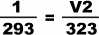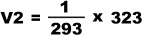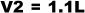### Gay-Lussac’s Law

Also known as the Third Gas Law, or Constant Volume Law

This states that if the volume remains the same, the pressure of a fixed mass of gas is directly proportional to the absolute temperature.

If the temperature of a fixed mass of gas is increased and the volume remains constant, then the pressure of the gas will increase.

Formulae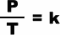(Where P is pressure, T is absolute temperature in Kelvin and k is a constant.)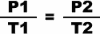(Where P1 and T1 are starting values, P2 and T2 are final values.)

Example

An air tank gauge shows a pressure of 2500psig at a temperature of 10°C – what is the pressure in the tank if the temperature rises to 40°C? Firstly, start temperature is 283K (as -273K is absolute zero) and final temperature is 313K. Absolute pressure is 2500 + 14.7psi (standard pressure of atmosphere).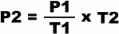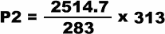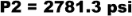(-14.7 for gauge pressure)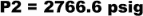The relationship between the temperature and pressure of a gas is an important consideration when using compressed gas in a fixed volume container, such as a SCUBA tank left in direct sunlight.

### General Gas Law

By combining several gas laws the relationship between the pressure, volume and temperature of a gas can be examined if one or more of these factors is changed.

Formula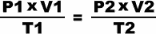(Where P1, V1 and T1 are starting values, P2, V2 and T2 are final values.)

Remember that when using the formula, absolute values should be used – i.e. temperature should be represented as Kelvin, where 0K (absolute zero) equals -273°C.

### Dalton’s Law

Every gas in a mixture of gases exerts its own partial pressure independently of the others. The pressure of a single gas in a mixture is known as the ‘partial pressure’.

Formula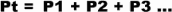(Where Pt is total pressure, P1 is the partial pressure of gas one, P2 of gas two, etc.)

Example

The simplified composition of air is 21% oxygen and 79% nitrogen. If the total air pressure equals 1bar, then the partial pressure of the two gases will be 0.21bar and 0.79bar respectively – i.e. 21% of 1bar equals 0.21bar.

Partial pressure can be represented in any appropriate measurement unit. The partial pressure of oxygen (for example) is denoted as pO2.

The partial pressures of oxygen, nitrogen and carbon dioxide are of particular relevance when discussing human physiology.

### Henry’s Law

When a liquid is exposed to a gas, some of the gas molecules will dissolve into it. The number of molecules that dissolve into the liquid depends on the mass of the liquid and also the partial pressure of the gas, its solubility and the surface area of contact.

Oxygen is normally carried by haemoglobin (red blood cells) and only dissolves into the liquid component of blood in small amounts. If the partial pressure of the oxygen is increased, as with hyperbaric therapy or diving, then more oxygen molecules will dissolve.

Unfortunately, this is also the case for other gases in the atmosphere – including nitrogen. Because nitrogen is an inert gas not utilised by the body, it will accumulate in the tissues until they can absorb no more molecules at that pressure.

As pressure is reduced, the nitrogen in the tissues is carried away only slowly by the blood to the lungs where it can leave the body. The nitrogen can be forced out of the tissues more quickly by breathing an oxygen-enriched atmosphere.

### Hyperbaric Oxygen Therapy

Because of the similarity between diving chambers and therapy chambers, diving terms and requisites are often used in hyperbaric oxygen therapy – e.g. chamber pressure is referred to as depth and treatment sessions are called dives.

The patients receiving HBO therapy can be divided into two groups –

• Those who benefit from the recompression and extra oxygen available to them while under pressure.
• Those who benefit solely from the extra oxygen available to them while under pressure.

The first group are almost exclusively recreational SCUBA divers suffering from decompression illness (DCI) caused by the formation of nitrogen bubbles in the body. See the SCUBA diving section for information on diving and decompression illness section for more information on DCI.

The second group benefit from the fact that while under pressure there are more molecules of oxygen available to the body’s cells. For these patients the treatment is either for carbon monoxide poisoning or for wounds or infections that respond well to hyperbaric oxygen therapy.

Gas Laws Formulas & Physics For Scuba Diving